## 9.4 Finishing with a Pretty Picture of a Fractal Equation

We’ve been around the block and then some. In these many Notebooks we’ve examined a plethora of concepts and equations, framed with a multiplicity of letters formed into words. As a Right Brain relief we are ending this sequence of Notebooks with a visual representation of a Fractal Equation - Nothing new theoretically - Just a Pretty Picture.

### A. The first round of simplifications with some new conventions

After so much extraneous verbiage, let us take this opportunity while creating our visualization to review where we’ve been so that we can make sense of what follows.

#### Decaying Average

Long ago, back at what seems to be the beginning of time we started with the Decaying Average. This is a measure of central tendency, which is based around decay and applied to the Live Data Stream. Below is its basic formula.In words, the Decaying Average of the Nth point in the Data Stream is equal to the most recent Data Point divided by the D, the Decay Factor, added to the past Decaying Average, which has been diminished by a fixed ratio, (D-1)/D, which we will call K.

It was speculated that all animate creatures use the Decaying Average to predict the most probable outcome. It was then speculated that many of these moving creatures also calculated the Deviation to predict the expected Range of Variance of the most probable outcome and the Directional to predict the Tendency of the Range of Variance.

#### The Higher Derivatives

Both of these are Higher Derivatives of the Decaying Average. Hence we referred to the Decaying Average as the Primary Derivative of the Data, while the Deviation and Directional were referred to as Secondary Derivatives. While these equations are Derivatives of the Decaying Average, it was seen that Derivatives of Derivatives could also be generated. For this reason we also called these the First Deviation and the First Directional of their respective series. Below are their equations.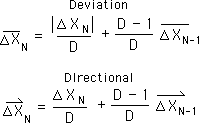Where#### Higher Derivatives of Directionals our focus

Notice that the Deviations are a scalar series, where the direction of change is irrelevant. The Directionals, on the other hand, are vectors that also acknowledge the direction of the change. It is the Directional Series of Derivatives that will eventually yield our Pretty Fractal Picture. We introduced the following notation to conveniently refer to the higher Directional Derivatives. Note that the Decaying Average is the Zeroth Directional.#### Our inner Hard Scientist wants a Content based equation

Notice also that all these definitional equations that we have introduced for the Decaying Average, Deviations and Directionals are all contextual, in that they are based upon what went immediately before. The hard scientist inside was a little uncomfortable with this soft approach and wanted to return to the real data - He wanted a content-based equation to replace these context based equations. Following is the Equation he derived for the Decaying Average.Where#### The Furling Function

The Contextual Scientists began laughing at this cumbersome expression.

The Deductive Scientist said, “No problem. Here is a notational short cut. I am introducing the Furling Function for simplification. Plus it has a cool spiral symbol to represent its coiling action. Here it is.#### The Fractal Root Pattern

In this way we can write our content based equation for the Decaying Average in a the simplified form shown below.”This relation forms the foundation of our system of derivatives. It is the building block - the Fundamental Element. Because of its scaling feature, it has been called the Fractal Root Pattern.

#### Circle Notation

The contextual scientists were somewhat chagrined and embarrassed at the success of the hard scientist with his laws and his insistence on raw data. “How about the First Directional. What are you going to do with that? Huh? You are going to come up with a real mess now.”

The Deductive Scientist went to work and came up with the following.

“First another notational simplification. We substitute Circle N for XN, so we don’t have so many subscripts. Below we summarize our relations.”#### A verbalization just to appreciate symbolic notation

Let us verbalize what this mess means just to appreciate symbolic notation. The first term, X Bar of N, is the Decaying Average at the Nth point of the Data Stream. This is also the same as the Zeroth Directional represented by Circle Zero of N, which is the second term of the equation. The third term is the content-based approach to determining this quantity, i.e. the Decaying Average or the Zeroth Directional of XN. To retrieve this value it would be necessary to unfurl the whole Data Stream, starting with X of N or XN and then continuing until X1 and then dividing the whole unfurling by D, the Decay Factor. The procedures for unfurling have been set out in previous pages and have no relevance to the pictures/equations we are about to generate. We will just mention that it has to do with the summation of successive members of the Data Stream, which have been scaled by the Konstant, traditionally called K, which equals (D-1)/D. The fourth term of the equation is just a notational variation where Circle N has been substituted for XN. While N represents the Number of the Data Point in the Stream, Circle N represents the actual value of the Nth Data Point.

#### A Furled equation for the 1st and 2nd Directionals

Based on these conventions we can write a content-based equation for the first Directional that fits on this page. Shown below.Contextual: “That’s pretty good, but how about the Second Directional.”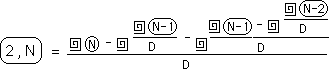#### Raveled Data or the expanded Circle Convention

Contextual: “It’s all right, but it’s getting real complex.”

Content: “OK then. Here is another notational simplification that will help out. I call it Raveling the Data under the circle notation. Circle squared yields the Zeroth Directional, i.e. the Decaying Average.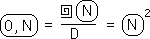#### 1st and 2nd Directionals as Raveled Numbers

The First Directional is shown below using the circle notation.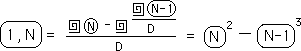And the Second Directional with the circle notation:#### The Remainder notation

To confuse and simplify things and for the sake of our picture we introduce another notational simplification, the Remainder notation, previously developed.With this notation Circle 0 is substituted for Circle N, i.e. XN, the Nth Data Point in the Stream. Under this context Circle 0 is the Data Point of the Now. In a similar fashion the Rth Point before the Now, Circle 0, is represented by Circle R, rather than Circle N-R or XN-R. This saves our eyes from a tremendous number of redundant Xs and N-1’s. These symbolic reductions allow us to recognize patterns more easily.

#### The Decaying Average as concentric circles

Using this notation we have the following equality for the Decaying Average of the Nth point or the Now point, i.e. the 0 point.Note that at the end of this equality we introduced the double circle to represent circle squared - Not so good for computational clarity but good for our fractal picture as we shall see.

#### First Directional in concentric circles notation

With this new simplexity we can now move on to the next layer. Below is the content-based equation for the First Directional at the Nth Point in the Data Stream, Circle 1 of N.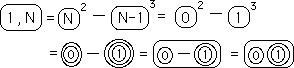The second and third expressions represent our equations as raveled numbers with the circle notation: first with a base of N and second with the remainder notation. The first expression of the second line is the same equation written with real circles rather than exponents. The second expression of the second line joins the external circles of the two raveled numbers. The final expression eliminates the negative sign as a convention. It also represents the beginning of our pretty picture.

#### The subtraction convention

The convention is that the circled expression on the right is subtracted from the one on the left, just like in normal subtraction. We are omitting the subtraction sign purely for the sake of our pretty picture. The subtraction sign is understood just like a 1 in front of the Variable X. We are choosing this convention for economy of representation, which is certainly linked with aesthetics.

#### A feeble attempt at a Verbal description

Another verbalization to appreciate the symbolic approach: each circle represents an unfurling of the Data starting with specified Number of the Data Point in the Stream. The first circle unfurls the Number of the Data Point into the Data Point itself. The second circle unfurls the Data Point into a Decaying Average of the Nth Data Point. The third Circle further unfurls the Decaying Average into a Raveled Number. The difference between the Present Data Point raveled once and the Past Data Point raveled twice, i.e. the difference between the Decaying Average of the Present Data and the First Directional of the Past Data is the First Directional of the Present Data. Glad we have symbols. God bless algebra.

#### The Second Directional as concentric circles

And finally the Second Directional with this visual circle notation. Below is a rough picture of the Second Directional. The same conventions that we have developed are followed. The second expression of the first line is the content-based expression in raveled notation. Remember that each Circle after the first represents an unfurling and a division by D, the Decay factor of the chain of data, i.e. the Data Stream, signified by the Number. This is a single unraveling. The exponent less one represent the number of times each piece of data needs to be unraveled.The expressions in the second line of the equation use the circle abbreviations previously introduced. First circles replace the exponents. Then the external circles of the numbers are joined. Next the subtraction symbol is removed. To reverse the process each circle except the innermost represents an unraveling and the circled numbers of the right are subtracted from those on the left. We’ll see an example of this process a little later on.

#### Circle acts as parentheses

Note: The circle which join numbers together as a group acts likes a parenthesis for the negative sign. For instance while the 2 is subtracted from the 1 before it in the second expression, it is added to the 0 Circle of the first expression. {For completion this should be proved, but for now we will just assert its truth, knowing it to be true.}

#### Verbalization for torture

In words, just for torture, this equation states that, as we’ve shown previously, that the Second Directional is equal to the ‘Present Data Raveled Once’ minus ‘the Previous Data Raveled Twice’, ‘this entire quantity’ minus the ‘next entire quantity’, which is the ‘Previous Data Raveled Twice’ minus the ‘Data Before That Raveled Thrice’. If you understand that garble, you are the only one in the entire universe. How does it feel to be so unique?

#### The Third Directional

Following a similar process for the third Directional we see the traditional equation below.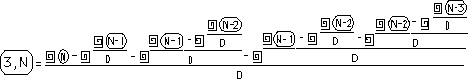From this equation, we move easily to the circle notation shown below.Remember that each circle represents a raveling of the Data from the ordered number shown that is circled. Remember also that the raveled numbers on the right are subtracted from those on the left.

#### The Fourth Directional

Below is the fourth Directional shown in circle form. As the pattern has established itself, we went directly ahead rather than fiddling with any intermediate equations.Note that each of the previous Directionals is contained in the whole. For instance the 3rd Directional is the first half of the equation, while the 2nd Directional is the first quarter, the 1st Directional is the first eighth, and the Zeroth Directional is the first term of the 16 terms which make up the fourth Directional.

### B. More simplifications and a prettier picture

In the above equations we still have numbers, which should not really be in a pretty picture. Furthermore our line of numbers is doubling with each new Directional. Without some serious scrunching, the fifth Directional will not even fit on the page. With these limitations in mind we introduced some more simplifications.

#### The Zeroth Directional with no numbers

We start with the Zeroth Directional, i.e. the Decaying Average of XN, by eliminating the numbers and just leaving the circles.This represents the Decaying Average, which every Derivative is tied to.

#### The First Directional revisited, number convention

Below is the 1st Directional without numbers.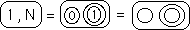Roughly speaking the new convention is that the circles on the right are always one more than the one on the left when dealing with the Remainder Notation, while vice versa with the N Notation.

#### The 2nd Directional revisited, below subtracted from above

Below is the 2nd Directional without numbers. A new convention is added. The circles below are subtracted from the ones above and the numbers below are one more than the numbers above.#### The 3rd Directional revisited

Below is the 3rd Directional without numbers. The same subtraction convention holds, i.e. subtract right from left and subtract bottom from top. Our number convention is modified to read that the numbers of the greater circles are always one more than the previous numbers. Or put another way the number inside the inner circles is always two less than the number of lines surrounding it. For instance there are five circular lines separating the bottom right circle from the outside so the number placed here should be 5 -2 =3.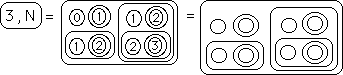#### The 4th Directional revisited

Below is the 4th Directional without numbers. The Subtraction and Number Conventions both hold. Subtract right and bottom from left and top. The number in the inner circle equals the number of lines that separates it from the outside minus 2. (Remember that we are using the Remainder Notation where we start in the Present, represented by 0 and then move into the past, represented by the positive integers starting with 1.)### C. An Old Friend: the square notation applied to Picture

#### Breaking down the picture

Let’s have a little fun, by reversing the process and reintroducing some old characters with a new twist. First we start with the artistic representation of the Fourth Directional of XN. To use this version we must first return to the numbered version shown below. Then we break the external ovals apart and add the circles back on to the smaller unit. Simultaneously we replace the minus signs that were implied in the above convention.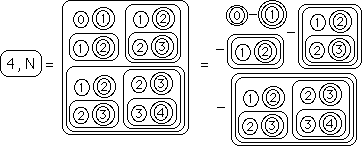#### Circles reversed into Exponents and negative signs

The next stage is to convert the visual circles into exponents. Simultaneously we broke apart some more of the larger ovals, circling the smaller units and replacing the implied minus signs, which sometimes turned into a positive sign if there was already a negative sign preceding the expression.This same process is continued in the next equation. More exponents are added and the larger ovals are further broken down replaced with smaller circles and minus signs.And then the final exponentiation and negative of the inner circles: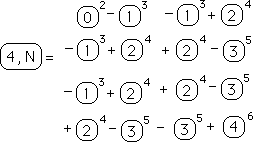We combine like terms.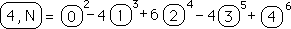#### The square notation returns

We reintroduce the square notation, which is another simplification to reduce the number of the exponent.In case you don’t remember this is just another representation of our Fractal Root Equation shown below.#### Another convenient aspect of the square notation

Another aspect of the square notation is that with each additional number an additional layer of raveling is added on. This is shown below.Using this Square Notation instead of the circle notation our equation for the 4th Directional becomes:According to derivations developed earlier this is equal to:which is a more specific version of the general equation for the P th Directional at the Nth point in the Data Stream. This confirms the validity of our Pretty Fractal Picture Equation and reintroduces our old friend, the square notation with the weird multiplication properties.#### Square Notation for Raveled Data, Circles for Raw Data

Remember that the square notation is just another way of representing a raveled number. While it is more direct than the circle notation, the square notation is unable to express the raw data in a simple way, while for the circle notation it is represented by a circled number. Possibly one could represent the numbers without a square and apply certain conventions, but that could lead to some incredible misunderstandings. This is why the circle and square notations are both needed to represent the raveled numbers.

### D. Our Old Friend, the Folding Function

#### The Folding Function in Square Notation

Remember our old friend the Folding Function, which eventually led to the famous Seed Equation, i.e. the General Equation the Pth Directional for the Nth Data Point based on content. If you don’t, you’re not alone. Here it is below.Converting to Remainder Notation yields the following.And then a further conversion to the square notation yields: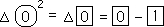#### Applying the Folding Function to our Pretty Picture

Below is the Numbered Picture of the Fourth Directional, which came from the Unnumbered Picture many pages earlier.Applying the Folding Function once yields:We just converted all the inner differences to the Square Folding Notation. What do we do with all of these folded squares? Use the following convention: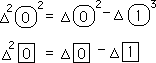Applying this procedure of double folding yields the following simplification:The double folding becomes a triple folding under the following process:Applying this folding yields the following:The triple folding becomes a quadruple folding under this process:#### The General Seed Equation for the Fourth Directional

Applying this folding yields the General Seed Equation for the fourth Directional. Shown below: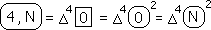It is shown in square notation, in circle notation with the Remainder notation, and with the N Notation. Notice that the Zero Square, which equals the Decaying Average, which is the Fractal Root Equation, only needs to be folded four times to yield the Fourth Directional. Simplicity itself. But the Process would be a nightmare. Complexity.

#### The General Seed Equation for the Nth Directional

This is in congruence with the General Seed Equation, shown below.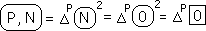Again we show the different notations with the folding function. Each of the circle and square elements represent the Decaying Average folded up P times. Remember also that the content based General Equation can also be written like this:This means that:This says that the Folding Function is directly linked with the Polynomial Expansion of Raveled numbers.

#### Thanks be to the Gods

We bow down before the glory of the Universe that could lead us to such simplicity in the midst of so much complexity. The fundamental nature of the Decaying Average is so great that it is the fundamental building block of all the Directionals. That the Gods would reveal this mystery is awesome. A Fractal Crystalline Structure that is revealed visually in the next few pages.

#### Non-verbal appreciation

The exploration in this section has reintroduced us to a few of our old friends, primarily the square notation of raveled numbers and the folding function. Then the gods led us to connect them up in this simple package. However the point of this whole section is aesthetic, not analytical. We are demonstrating the beauty of these Fractal Equations. The order, the symmetry, the irregularity is breath taking. Again the ability to appreciate the beauty is Divine. To sigh before the magnificent order of the Universe is a gift bestowed upon us tiny mortals by Forces far beyond our ken and understanding. Read on to see the complex aesthetics of these Fractal Equations. This appreciation does not come from the verbal side. To groan with delight over the order comes from the non-verbal.

### E. Back to the Pictures of the Higher Directionals

#### The 5th and 6th Directionals at last

Below are pretty pictures of the 5th and 6th Directional. They both follow the above conventions, which include the Circle, Subtraction, and Number conventions. Each Circle represents a Raveling of the implied Number inside the inner circles, which is determined by the number of lines from the outside minus two. Further right is subtracted from left and bottom from top.#### The 7th Directional

And a pretty picture of the 7th Directional, which follows all the same conventions, established above.#### An even prettier -picture of the 7th Directional#### The 7th Directional with a little more variation#### How about the 8th Directional, a little scrunched up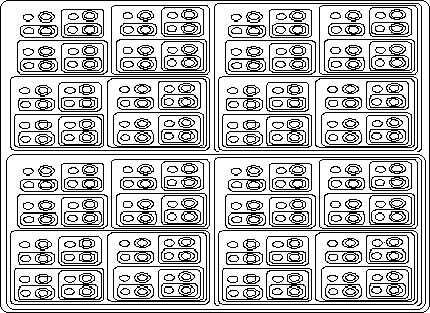#### The 9th, 10th, 11th and 12th Directionals, even more scrunched up

On the following two pages are the 9th through 12 Directionals scrunched enough so that they will fit on the page. Note again that each circle represents a raveled number, which must be unraveled and unfurled individually to yield the desired Directional. This computation can be quite complex depending upon how many numbers there are in the Data Stream and how many time the implied number is circled. Each circle represents a separate unraveling. Simply speaking the computations for just a single circle in these pretty pictures would be prohibitive if our Data Stream contained even 20 elements. Thus these equations are not for computation but only to look pretty.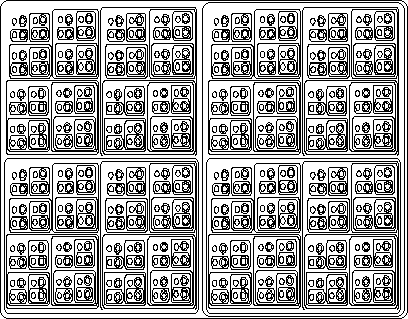#### Summary: Content versus context revisited a final time

Remember that these pretty pictures are just fractal equations of the Directionals. They are content-based equations whose complexity is visually apparent. In reality we use context-based equations to compute these Directionals. The context based equations ignore all the previous data, only retaining a few numbers, i.e. a few central measures which represent what went before and the new Data Point, which is represented by the small circle on the top left. While yielding the necessary information, the context-based equation doesn’t expose the fractal nature of the central measures, nor does it reveal such pretty pictures.